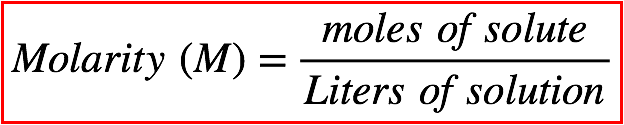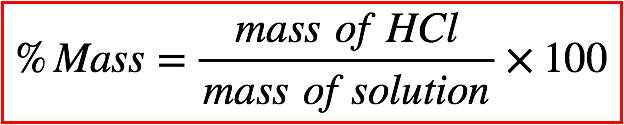# Problem: Commercial grade HCl solutions are typically 39.0% (by mass) HCl in water.  Determine the molarity of the HCl, if the solution has a density of 1.20 g/mL.A) 13.9 M      B) 12.8  M      C) 10.7 M      D) 9.35 M      E) 7.79 M

###### FREE Expert Solution
87% (256 ratings)
###### FREE Expert Solution

We’re being asked to calculate the molarity (M) of hydrochloric acid (HCl) in a solution. Recall that molarity is the ratio of the moles of solute and the volume of solution (in liters). In other words:We first need to determine the number of moles of HCl. We’re given the mass percent of HCl in water, 39.0%. Recall that mass percent is given by:87% (256 ratings)###### Problem Details

Commercial grade HCl solutions are typically 39.0% (by mass) HCl in water.  Determine the molarity of the HCl, if the solution has a density of 1.20 g/mL.

A) 13.9 M

B) 12.8  M

C) 10.7 M

D) 9.35 M

E) 7.79 M

What scientific concept do you need to know in order to solve this problem?

Our tutors have indicated that to solve this problem you will need to apply the Molarity concept. You can view video lessons to learn Molarity. Or if you need more Molarity practice, you can also practice Molarity practice problems.

What is the difficulty of this problem?

Our tutors rated the difficulty ofCommercial grade HCl solutions are typically 39.0% (by mass)...as medium difficulty.

How long does this problem take to solve?

Our expert Chemistry tutor, Dasha took 5 minutes and 24 seconds to solve this problem. You can follow their steps in the video explanation above.

What professor is this problem relevant for?

Based on our data, we think this problem is relevant for Professor Randles' class at UCF.